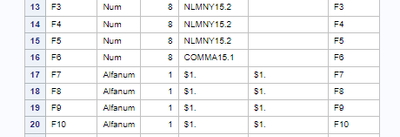## Meaning of "ERROR: Interval variables must be numeric (F7)."

Hi everyone,

I'm new to SAS studio and I was trying to use the "proc HPNEURAL" and after I specified all the parameters and the instructions, and ran the code, this message "ERROR: Interval variables must be numeric (F7)." was displayed in the LOG.

Someone can help me to understand what's the meaning of that and how to fix it?

If you need other information I'd glad to give it to you.

8 REPLIES 8

## Re: Meaning of "ERROR: Interval variables must be numeric (F7)."

You are trying to use a character variable where a numeric variable is required. You can't do that. Perhaps you need to change F7 to numeric first; or perhaps you simply don't want to change F7 to numeric, in which case you can't use it as an interval variable, but it could be used as a categorical variable. You have look at the values of F7 and decide how to move forward.

--
Paige Miller

## Re: Meaning of "ERROR: Interval variables must be numeric (F7)."First of all, thank you for your help!
I saw in the import output that F7 column is taken as alfanum, but in excel (input file) the cells type is "number" and the content is only the value 0 or 1.
How can I change the type of f7,F8 and ecc from Alfanum in Num?

## Re: Meaning of "ERROR: Interval variables must be numeric (F7)."

Save the Excel data to text (csv) and read that with a DATA step you write; this way you get full control over variable names, types, lengths and formats.

## Re: Meaning of "ERROR: Interval variables must be numeric (F7)."

"IMPORT"?

What is the source of the SAS dataset?  Did you use PROC IMPORT to read from a TEXT file, such as a CSV file?  If so then replace the PROC IMPORT step with your own data step where you will have control over how the variables are defined.

If you used PROC IMPORT to read from an actual EXCEL worksheet it would only have made F7 as character if the column was completely empty or there was at least one cell that had a character string instead of a number.

## Re: Meaning of "ERROR: Interval variables must be numeric (F7)."

Excel is always irrelevant. You need to check the SAS data set and not the Excel file, because SAS Studio works on SAS data sets, not Excel files.

I believe you might want to write simple code to do this and then work with the new variable F7A.

``````data new;
set old;
f7a = input(f7,1.);
run;``````

However ... this really isn't what HPNeural is expecting either. This is NOT an interval variable. 0/1 binary variables are not interval variables. Changing it to numeric doesn't make it an interval variable. You most likely want this as a nominal variable, in which case you can leave it as alphanumeric (I think).

--
Paige Miller

## Re: Meaning of "ERROR: Interval variables must be numeric (F7)."

Thank you very much.
How can I make F7,F8, ecc... a nominal variable instead of an interval variable?

## Re: Meaning of "ERROR: Interval variables must be numeric (F7)."

According to the HPNeural documentation, you create the proper INPUT statement(s) for Interval variables, and more INPUT statement(s) for the Nominal variables.

--
Paige Miller

## Re: Meaning of "ERROR: Interval variables must be numeric (F7)."

@DanielePugno wrote:First of all, thank you for your help!
I saw in the import output that F7 column is taken as alfanum, but in excel (input file) the cells type is "number" and the content is only the value 0 or 1.
How can I change the type of f7,F8 and ecc from Alfanum in Num?